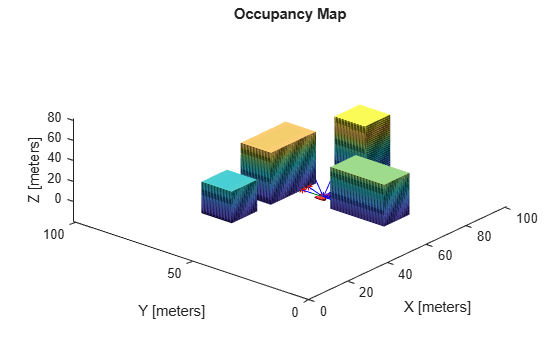# show

Show occupancy map

## Syntax

``axes = show(map3D)``
``show(map3D,"Parent",parent)``

## Description

example

````axes = show(map3D)` displays the occupancy map, `map3D`, in the current axes, with the axes labels representing the world coordinates.The function displays the 3-D environment using 3-D voxels for areas with occupancy values greater than the `OccupiedThreshold` property value specified in `map3D`. The color of the 3-D plot is strictly height-based.```
````show(map3D,"Parent",parent)` displays the occupancy map in the axes handle specified by `parent`.```

## Examples

collapse all

Import a 3-D occupancy map.

`map3D = importOccupancyMap3D("citymap.ot")`
```map3D = occupancyMap3D with properties: ProbabilitySaturation: [1.0000e-03 0.9990] Resolution: 1 OccupiedThreshold: 0.6500 FreeThreshold: 0.2000 ```

Inflate the occupied areas by a radius of 1 m. Display the map.

```inflate(map3D,1) show(map3D)```

Find the intersection points of rays and occupied map cells.

```numRays = 10; angles = linspace(-pi/2,pi/2,numRays); directions = [cos(angles); sin(angles); zeros(1,numRays)]'; sensorPose = [55 40 1 1 0 0 0]; maxrange = 15; [intersectionPts,isOccupied] = rayIntersection(map3D,sensorPose,directions,maxrange)```
```intersectionPts = 10×3 55.0000 32.0000 1.0000 57.9118 32.0000 1.0000 61.7128 32.0000 1.0000 67.9904 32.5000 1.0000 69.0000 37.5314 1.0000 69.0000 42.4686 1.0000 67.9904 47.5000 1.0000 64.6418 51.4907 1.0000 58.2757 49.0000 1.0000 55.0000 49.0000 1.0000 ```
```isOccupied = 10×1 1 1 1 -1 1 1 -1 -1 1 1 ```

Plot the intersection points and rays from the pose.

```hold on plotTransforms(sensorPose(1:3),sensorPose(4:end),... 'FrameSize',5,'MeshFilePath','groundvehicle.stl') % Vehicle sensor pose for i = 1:numRays plot3([sensorPose(1),intersectionPts(i,1)],... [sensorPose(2),intersectionPts(i,2)],... [sensorPose(3),intersectionPts(i,3)],'-b') % Plot rays if isOccupied(i) == 1 plot3(intersectionPts(i,1),intersectionPts(i,2),intersectionPts(i,3),'*r') % Intersection points end end```## Input Arguments

collapse all

3-D occupancy map, specified as an `occupancyMap3D` object.

Axes used to plot the map, specified as either an `Axes` or `UIAxes` object. See `axes` or `uiaxes`.

## Output Arguments

collapse all

Axes handle for map, returned as either an `Axes` or `UIAxes`object. See `axes` or `uiaxes`.